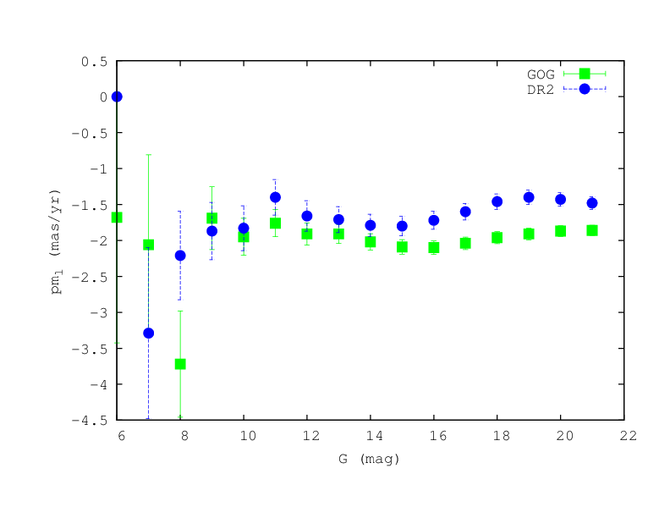# 10.3.2 Proper motion distributions

Proper motion distributions are computed for data (pre-DR2 release) and simulations separately, then combined and inter-compared. Absolute differences of the mean proper motions and of their standard deviations are done separately for $\mu_{l}*$ and $\mu_{b}$. Results for the mean proper motions are presented in following figures and tables, first as a function of magnitude, then over the sky.

Tables 10.1 (resp.  10.2) indicate the mean proper motion $\mu_{l}*$ (resp. $\mu_{b}$) over the whole sky per magnitude bins for model and data.

Figure 10.23 shows the mean proper motions ($\mu_{l}*$ and $\mu_{b}$) as a function of magnitude integrated over the whole sky. The differences are at the level of 0.2  mas yr${}^{-1}$ for stars brighter than 15 but increase at fainter magnitudes. It can be due to an inaccuracy of the model in the mean rotation for $\mu_{l}*$. On the contrary, for $\mu_{b}$ the difference is larger for bright stars and can reach a level of 1  mas yr${}^{-1}$ at magnitude less than 10. The overall change of mean proper motion with magnitude is very similar between the model and the data at magnitudes fainter than 12 with a difference of less than 30  $\mu$as yr${}^{-1}$ at $G>17$. Overall, it seems that the Gaia data are very good up to very faint magnitudes and it is probably the model which suffers from systematics.

At bright magnitudes (14-15), there is a generally good agreement between data and model, at the level of better than 0.5  mas yr${}^{-1}$ for both $\mu_{l}$ and $\mu_{b}$. At fainter magnitudes, the mean difference between data and model is larger.Figure 10.23: Mean proper motions in  mas yr-1 over whole sky as a function of magnitude (left: μl*, right: μb). Data are blue squares and model green circles.

Figures 10.24 ($\mu_{l}$) and 10.25 ($\mu_{b}$) show the distributions of the proper motions as a function of position on the sky for two magnitude ranges. It shows that the model reproduces roughly the distribution, with similar patterns in both distribution. However, there are systematics not well reproduced, as was already noted in the validation of Gaia DR1. It is most probable that the systematic differences are due to the model. We are waiting for the new GOG simulations which will use a model fitted on Gaia DR1 in order to better study if the problem could be due to the Gaia DR2 data themselves.Figure 10.24: Mean proper motions μl* in Gaia DR2 (left), in GOG simulation (med) and their absolute differences (right) in  mas yr-1 in HEALPix bins, in 2 magnitude ranges 12Figure 10.25: Mean proper motions μb in Gaia DR2 (left), in GOG simulation (med) and their absolute differences (right) in  mas yr-1 in HEALPix bins, in 2 magnitude ranges 12